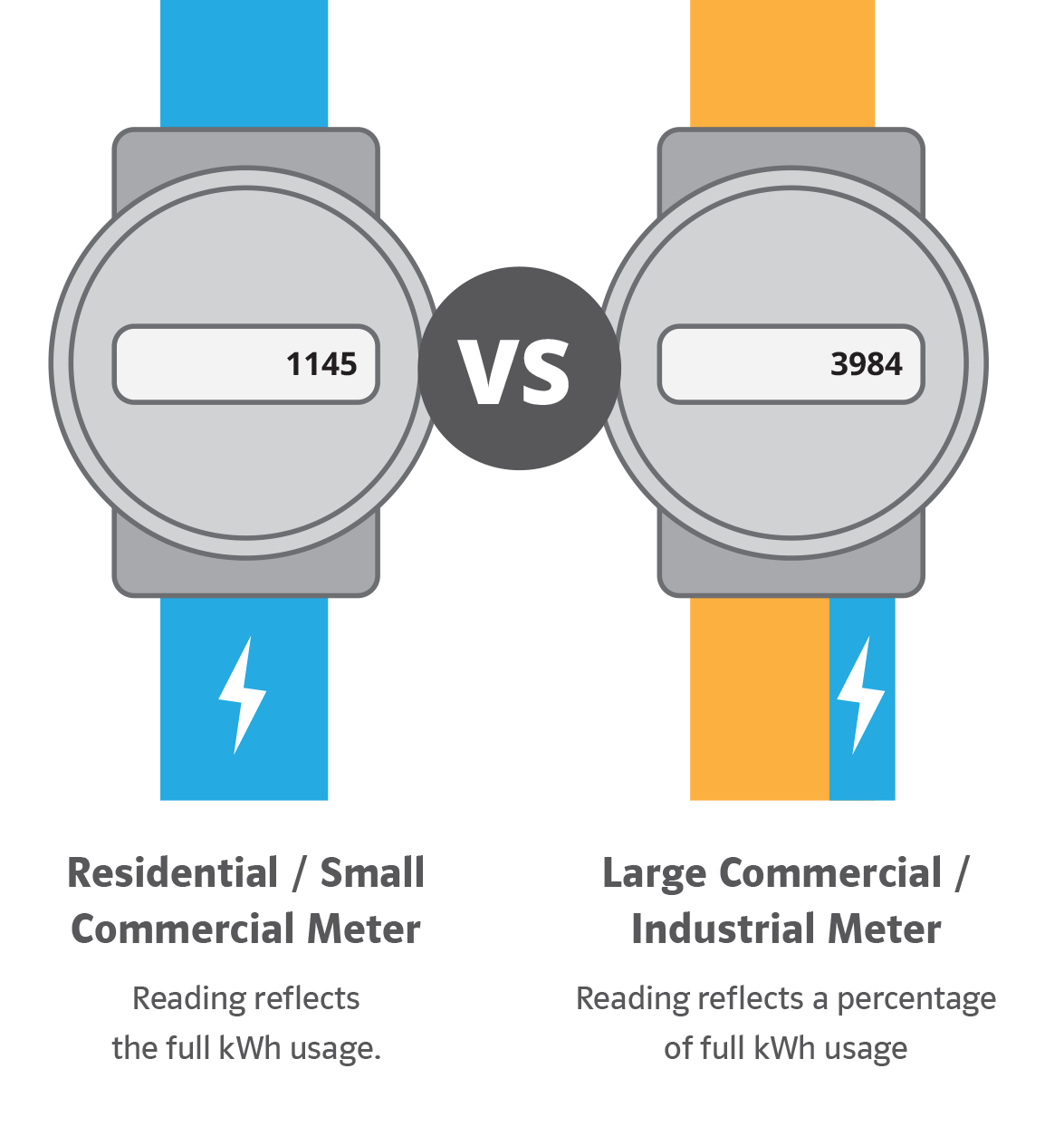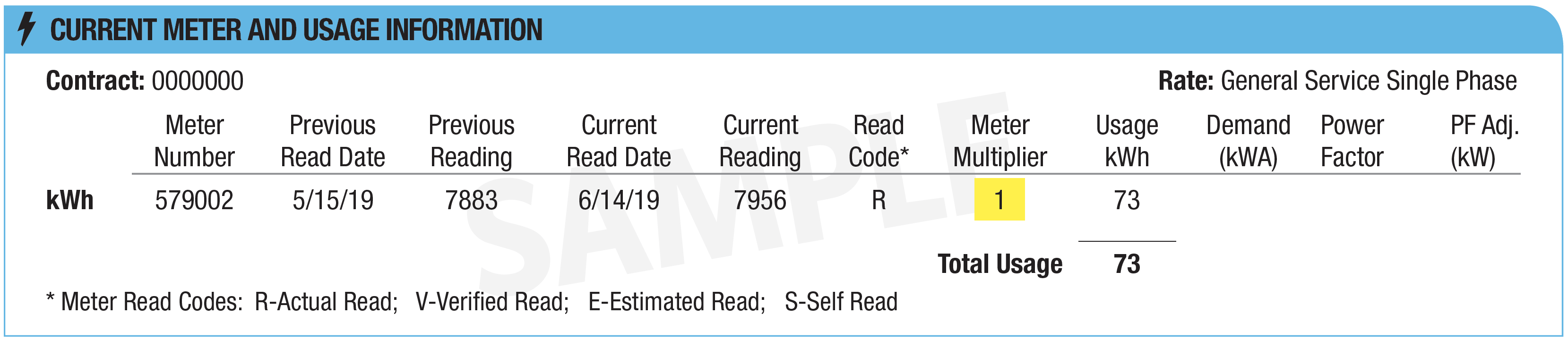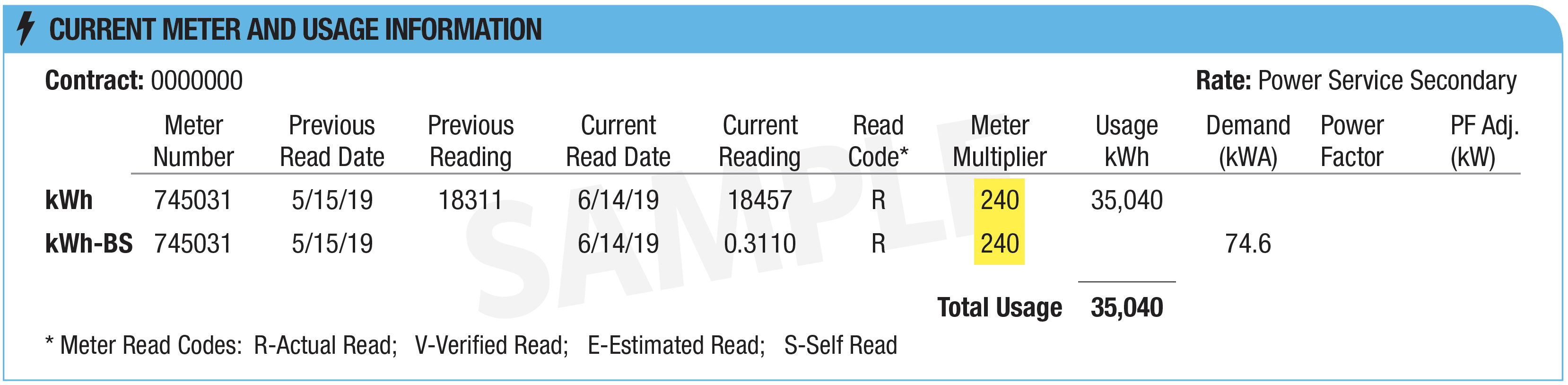Meter Multiplier

The actual voltage/current used is often too large to be registered by your meter. Therefore, a meter’s registering capacity may only represent a small percentage of your actual load. To determine the actual usage on the demand meter, the registered usage must be multiplied by the meter multiplier.Residential / Small Commercial Meter

Meter reading reflects the full kWh usage.

Large Commercial / Industrial Meter

Meter reading reflects a percentage of the full kWh usage.

The actual voltage/current used is often too large to be registered by your meter. This multiplier is determined by the efficiency of your infrastructure.

How is it calculated?

(Current Reading - Previous Reading) x Meter MultiplierReading reflects the full kWh usage.Reading reflects a percentage of the full kWh usage. Calculated by subtracting the previous reading from the current reading then multiplying by the meter multiplier.